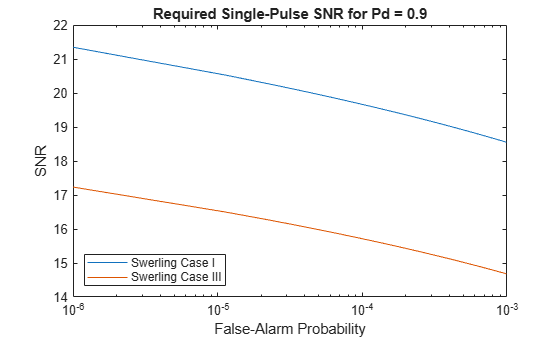Main Content

# shnidman

Required SNR using Shnidman’s equation

## Syntax

```SNR = shnidman(Prob_Detect,Prob_FA) SNR = shnidman(Prob_Detect,Prob_FA,N) SNR = shnidman(Prob_Detect,Prob_FA,N, Swerling_Num) ```

## Description

`SNR = shnidman(Prob_Detect,Prob_FA)` returns the required signal-to-noise ratio in decibels for the specified detection and false-alarm probabilities using Shnidman's equation. The SNR is determined for a single pulse and a Swerling case number of 0, a nonfluctuating target.

`SNR = shnidman(Prob_Detect,Prob_FA,N)` returns the required SNR for a nonfluctuating target based on the noncoherent integration of `N` pulses.

`SNR = shnidman(Prob_Detect,Prob_FA,N, Swerling_Num)` returns the required SNR for the Swerling case number `Swerling_Num`.

## Examples

collapse all

Find and compare the required single-pulse SNR for Swerling cases I and III. The Swerling case I has no dominant scatterer while the Swerling case III has a dominant scatterer.

Specify the false-alarm and detection probabilities.

```pfa = 1e-6:1e-5:.001; Pd = 0.9;```

Allocate arrays for plotting.

```SNR_Sw1 = zeros(1,length(pfa)); SNR_Sw3 = zeros(1,length(pfa));```

Loop over PFA's for both scatterer cases.

```for j=1:length(pfa) SNR_Sw1(j) = shnidman(Pd,pfa(j),1,1); SNR_Sw3(j) = shnidman(Pd,pfa(j),1,3); end```

Plot the SNR vs PFA.

```semilogx(pfa,SNR_Sw1,'k','linewidth',2) hold on semilogx(pfa,SNR_Sw3,'b','linewidth',2) axis([1e-6 1e-3 5 25]) xlabel('False-Alarm Probability') ylabel('SNR') title('Required Single-Pulse SNR for Pd = 0.9') legend('Swerling Case I','Swerling Case III',... 'Location','SouthWest')```The presence of a dominant scatterer reduces the required SNR for the specified detection and false-alarm probabilities.

## More About

collapse all

### Shnidman's Equation

Shnidman's equation is a series of equations that yield an estimate of the SNR required for a specified false-alarm and detection probability. Like Albersheim's equation, Shnidman's equation is applicable to a single pulse or the noncoherent integration of `N` pulses. Unlike Albersheim's equation, Shnidman's equation holds for square-law detectors and is applicable to fluctuating targets. An important parameter in Shnidman's equation is the Swerling case number.

### Swerling Case Number

The Swerling case numbers characterize the detection problem for fluctuating pulses in terms of:

• A decorrelation model for the received pulses

• The distribution of scatterers affecting the probability density function (PDF) of the target radar cross section (RCS).

The Swerling case numbers consider all combinations of two decorrelation models (scan-to-scan; pulse-to-pulse) and two RCS PDFs (based on the presence or absence of a dominant scatterer).

Swerling Case NumberDescription
0 (alternatively designated as 5)Nonfluctuating pulses.
IScan-to-scan decorrelation. Rayleigh/exponential PDF–A number of randomly distributed scatterers with no dominant scatterer.
IIPulse-to-pulse decorrelation. Rayleigh/exponential PDF– A number of randomly distributed scatterers with no dominant scatterer.
IIIScan-to-scan decorrelation. Chi-square PDF with 4 degrees of freedom. A number of scatterers with one dominant.
IVPulse-to-pulse decorrelation. Chi-square PDF with 4 degrees of freedom. A number of scatterers with one dominant.

## References

 Richards, M. A. Fundamentals of Radar Signal Processing. New York: McGraw-Hill, 2005, p. 337.

## See Also

Introduced in R2011a

## Support

#### Exploring Hybrid Beamforming Architectures for 5G Systems

Download white paper